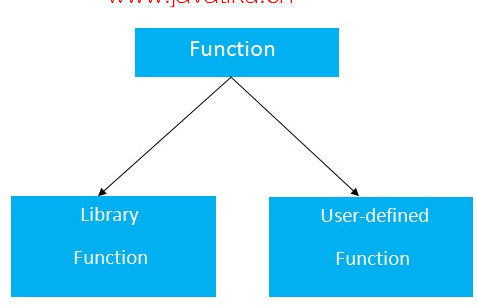# C++教程-C++函数## C++函数的优点

• 代码重用：通过在C++中创建函数，可以多次调用它。因此，我们不需要重复编写相同的代码。
• 代码优化：函数使代码变得优化，我们不需要编写太多的代码。

## 函数的类型

C语言中有两种类型的函数：

1. 库函数

1. 用户自定义函数## 函数的声明

C++语言中创建函数的语法如下：

``````返回类型 函数名(参数的数据类型...)
{
//要执行的代码
}``````

### C++函数示例

``````#include <iostream>
using namespace std;
void func() {
static int i=0; //静态变量
int j=0; //局部变量
i++;
j++;
cout<<"i=" << i<<" and j=" <<j<<endl;
}
int main()
{
func();
func();
func();
}  ``````

``````i= 1 and j= 1
i= 2 and j= 1
i= 3 and j= 1``````